• R语言对带有绝对值符号的函数求偏导并输出导数结果 求偏导后的导数表达式网上已有众多教程，这里主要对导数结果的输出及含有绝对值符号的的函数偏导运算进行方法阐述 ...#构造求偏导函数 fun = functio
R语言计算带有绝对值符号的函数偏导并输出导数结果
求偏导后的导数表达式网上已有众多教程，这里主要对导数结果的输出及含有绝对值符号的的函数偏导运算进行方法阐述
对函数f=x*|y|*(z-x)求对x、y、z的偏导，输出在点（1,2,3）和（1,-2,3）的导数值，代码如下：
#当y>0时，函数f如下：
fpo<-expression(x*y*(z-x))
#当y<0时，函数f如下：
fne<-expression(x*(-y)*(z-x))
#构造求偏导函数
fun = function(x,y,z){
if(y>=0){
a = eval(deriv(fpo,c("x","y","z")))
return(re)
}
if(y<0){
b = eval(deriv(fne,c("x","y","z")))
return(re)
}
}
#代入（1,2,3）检验结果
fun(1,2,3)
#代入（1,-2,3）检验结果
fun(1,-2,3)

结果如下：

fun(1,2,3)
x y z
[1,] 2 2 2
fun(1,-2,3)
x  y z
[1,] 2 -2 2


展开全文R语言
• 和，差，积，商的微分公式我们现在重新引入此符号的原因是我们不仅要接受与变量X有关的导数，还需要其它更多变量的导数，所以需要一些明确的符号来区分哪个变量现在假设我们有一个同时依赖于X和Y的函数，我们将其...
我们在一元微积分中对如下的微分算子都很熟悉：和，差，积，商的微分公式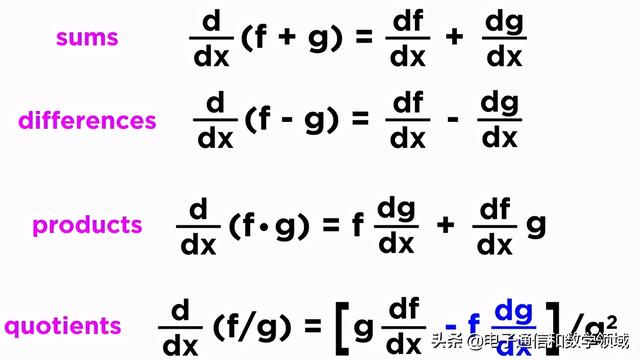我们现在重新引入此符号的原因是我们不仅要接受与变量X有关的导数，还需要其它更多变量的导数，所以需要一些明确的符号来区分哪个变量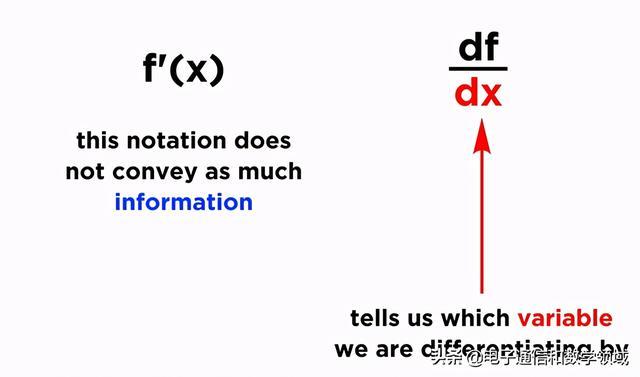现在假设我们有一个同时依赖于X和Y的函数，我们将其称为f(x,y)，这最终将称为曲面而不是曲线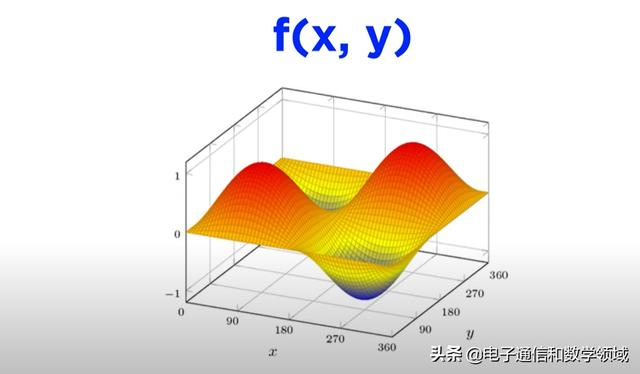那么这个曲面的变化率是多少，我们要做的就是找到它的变化率，如果想要找到X的变化率，我们仅将Y作为常数，以此区分作为变量的X，我们使用弯曲的D代替常规的D，这称之为偏导数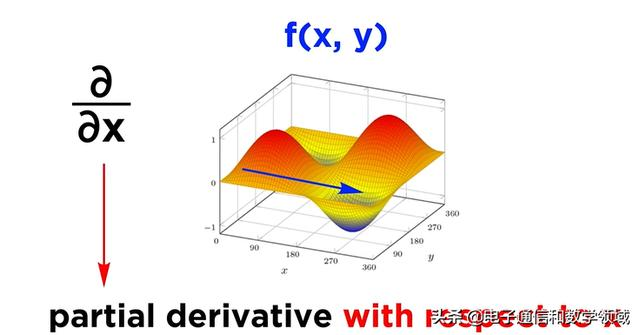同样，如果我们想要Y方向的变换量，这将是关于y的偏导数，在这种情况下，我们会将X视作常数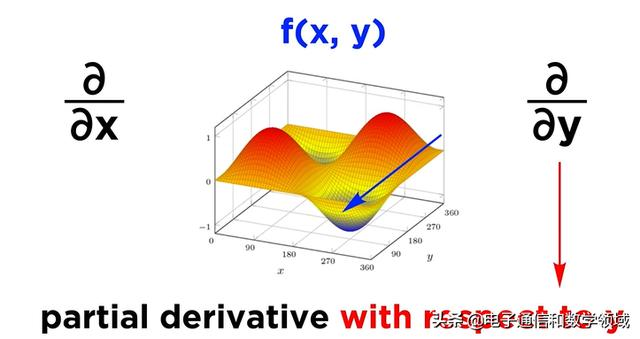如下是一个示例：关于X和Y的偏导数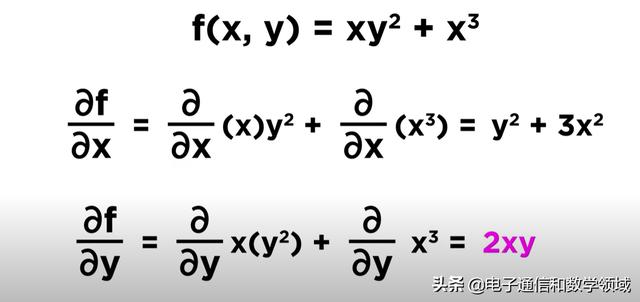现在，我们将介绍一个结合了这些偏导数，即所谓的梯度，函数的梯度只是一个向量，它有所有的偏导数组成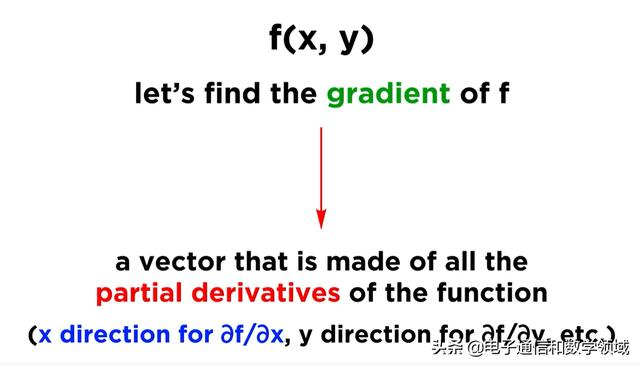我们可以写成gradf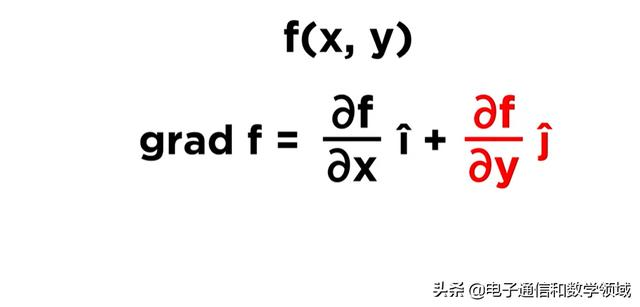如果在三个维度上，梯度就是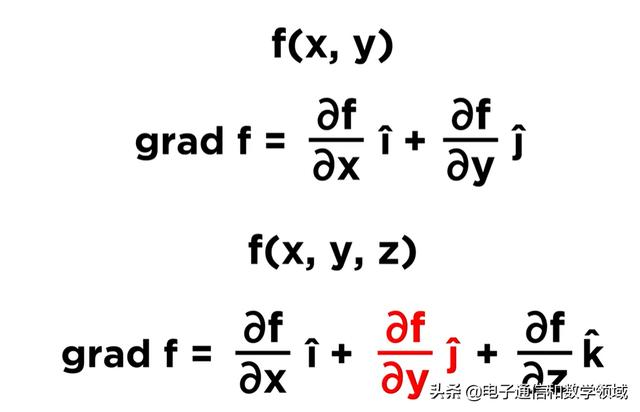这个向量它指向函数最大变化的方向，并等于最大变化率的幅度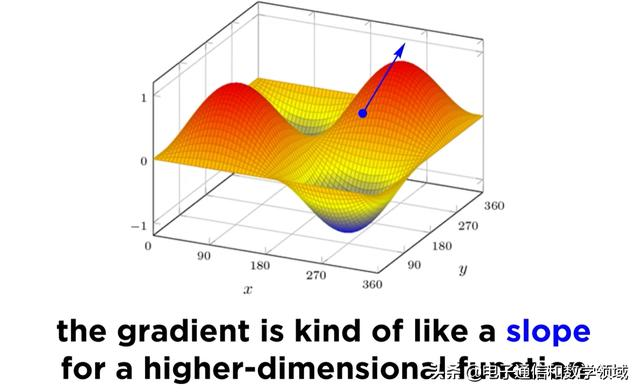这个有关向量的偏导数，即梯度用倒置的三角形表示，称为del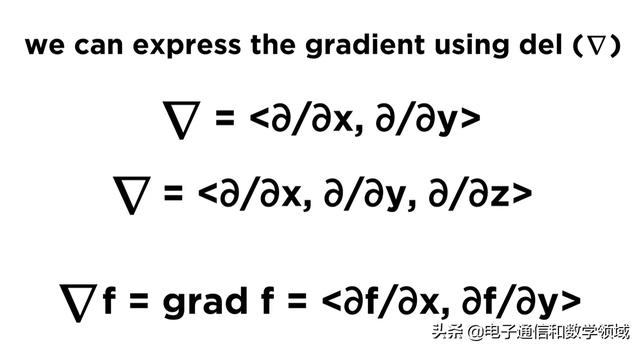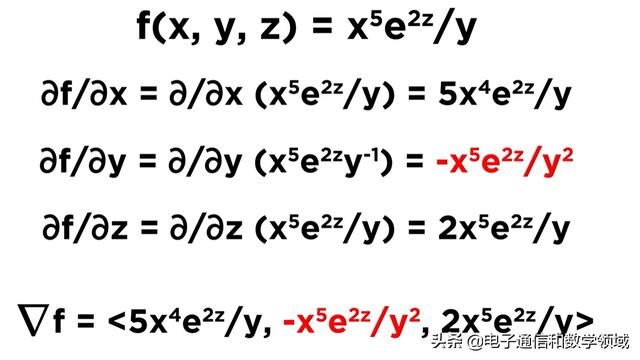展开全文• 【Sage数学库】符号计算：函数求导、求积分 帮助链接： https://doc.sagemath.org/html/en/reference/calculus/sage/calculus/tests.html # derivative 求导 print(derivative(arctan(x), x)) # 或者 diff 求导 ...
【Sage数学库】符号计算：函数求导、求偏导数、求积分

帮助链接：

https://doc.sagemath.org/html/en/reference/calculus/sage/calculus/tests.html

# derivative 求导
print(derivative(arctan(x), x))
# 或者 diff 求导
print(diff(arctan(x), x))

#求导
print(diff(-cos(x), x))
# 不定积分
integral(sin(x),x)
print(integral(sin(x),x))
y = var('y')
print(integral(sin(x),y))

# 定积分
# 定积分（上下限是变量）
var('a, b')
integrate(sin(x), x, a, b)
print(integrate(sin(x), x, a, b))

# 下面的定积分值 是 1
integrate(sin(x), x, 0, pi/2)
print(integrate(sin(x), x, 0, pi/2))

1/(x^2 + 1)
1/(x^2 + 1)
sin(x)
-cos(x)
y*sin(x)
cos(a) - cos(b)
1

下面求函数的偏导数：

参考： https://doc.sagemath.org/html/en/reference/calculus/sage/calculus/calculus.html

# ★★★★★★★★★★★ 【实际上，diff是用来求偏导数的！】 ★★★★★★★★★★
# https://doc.sagemath.org/html/en/reference/calculus/sage/calculus/calculus.html
f(x,y)=x^2*y+y^2+y
print("二元函数f(x,y)：")
print(f)
print("二元函数f(x,y)的【偏导数】：")
print(PianDaoShu_gradient)

二元函数f(x,y)：
(x, y) |--> x^2*y + y^2 + y
二元函数f(x,y)的【偏导数】：
(x, y) |--> (2*x*y, x^2 + 2*y + 1)

高阶偏导数：

# ★★★★★★★★★★★ 【实际上，diff是用来求偏导数的，而且可用求 高阶偏导数！】 ★★★★★★★★★★
# https://doc.sagemath.org/html/en/reference/calculus/sage/calculus/calculus.html
f(x,y)=x^2*y+y^2+y
print("二元函数f(x,y)：")
print(f)
print("二元函数f(x,y)的【偏导数】：")

# 对 x求 二阶偏导数
f_x_2_PianDao = f.diff(x, 2)
print("对 x求 二阶偏导数：")
print(f_x_2_PianDao)

# 对 y求 二阶偏导数
f_y_2_PianDao = f.diff(y, 2)
print("对 y求 二阶偏导数：")
print(f_y_2_PianDao)


二元函数f(x,y)：
(x, y) |--> x^2*y + y^2 + y
二元函数f(x,y)的【偏导数】：
(x, y) |--> (2*x*y, x^2 + 2*y + 1)对 x求 二阶偏导数：
(x, y) |--> 2*y
对 y求 二阶偏导数：
(x, y) |--> 2

备忘（有时间再实践）：

Sage里面的 泰勒公式？ ===>  taylor  ?

https://doc.sagemath.org/html/en/reference/calculus/sage/calculus/calculus.html

taylor(u(x+h),h,0,4)
1/24*h^4*diff(u(x), x, x, x, x) + 1/6*h^3*diff(u(x), x, x, x) + 1/2*h^2*diff(u(x), x, x) + h*diff(u(x), x) + u(x)


展开全文• 偏导数partial derivative 利用Sympy库 SymPy是一个符号计算的Python库。它的目标是成为一个全功能的计算机代数系统，同时保持代码简洁、易于理解和扩展。它完全由Python写成，不依赖于外部库。 SymPy支持符号计算...
求偏导数partial derivative
利用Sympy库
SymPy是一个符号计算的Python库。它的目标是成为一个全功能的计算机代数系统，同时保持代码简洁、易于理解和扩展。它完全由Python写成，不依赖于外部库。
SymPy支持符号计算、高精度计算、模式匹配、绘图、解方程、微积分、组合数学、离散数学、几何学、概率与统计、物理学等方面的功能。
程序代码
>>> from sympy import symbols, diff
>>> x, y = symbols('x y', real=True)
>>> diff( x**2 + y**3, y)
3*y**2
>>> diff( x**2 + y**3, y).subs({x:3, y:1})
3

先将所求变量（x，y）符号化。否则会提示为定义错误：NameError: name 'y' is not defined。之后利用diff函数求对应函数偏导数。
求出偏导数之后，若想求具体的值，可利用subs属性进行变量的替换，便可自动求出对应值。
参考链接：
https://docs.sympy.org/latest/index.html


展开全文python
• 偏导符号是圆体字母，区别于全导数符号的正体。 由定义可求得：   几何含义：偏导数f'x(x0,y0)表示固定面上一点对x轴的切线斜率；偏导数f'y(x0,y0)表示固定面上一点对y轴的切线斜率。   例...
• 提要  转行搞科研之后，发现最重要的是数学和物理。  偏导，方导，梯度，散度，旋度是高等数学的几个基本概念，在图形学中会经常用到，这里重新来学习一下，同时也当作一个记录。...偏导符号是圆体字算法 数学
• 偏导符号是圆体字母，区别于全导数符号的正体。 由定义可求得： 几何含义：偏导数f'x(x0,y0)表示固定面上一点对x轴的切线斜率；偏导数f'y(x0,y0)表示固定面上一点对y轴的切线斜率。
• 偏导 定义：一个多变量的函数偏导数是它关于其中一个变量的导数，而保持其他...偏导符号是圆体字母，区别于全导数符号的正体。 由定义可求得： 几何含义：偏导数f’x(x0,y0)表示固定面上一点对x轴的切线斜...机器学习
• 求导以及求偏导运算在数学中是很重要的一个部分，尤其是在高等数学中，基本都由函数的导数与偏导组成，很多公式定理也是关于这方面的，如果少了这一部分，数学将会黯然失色。因此在文档中涉及到这些内容时，必然会...
• 1 ，欧式空间 ： 学名 ： 欧几里得空间 解释 ： n 维实数空间 ( 对二维，三维空间的扩展 ) 2 ，超级函数组 ：(m,n) ...超级偏导数 ： 超级方程组的偏导数 雅克比矩阵 ： 就是 ( 超级偏导数 ) 符号表示
• 在一元函数中，我们已经知道导数就是函数的变化率。对于二元函数我们同样要研究它的“变化率”。 　在xOy平面内，当动点由P(x0,y0)沿不同方向变化时，函数f(x,y)的变化快慢一般说来是不同的...　偏导数的表示符号为...等高线
• 符号定义：{xi,i=1,2,⋯,n}是n个样本组成的样本集合，c为预定的类别数目，μi,i=1,2,⋯,c每一个聚类的中心，μj(xi)是第i个样本对于第j类的隶属度函数，且其满足例如以下关系式： ∑j=1cμj(xi)=1i=1,2,⋯,c(1) 用...
• Latex tips： \frac{}{}:分数表示 \partial: 偏导符号 \mathrm{d}t:正规的导数d（正体） 绘画转latex
• 目录一元函数的导数MATLAB函数语法应用举例例1 ：普通函数求导例2 ：复合泛函求导例3 ：矩阵函数求导多元函数偏导数MATLAB函数语法应用举例例1 ：求偏导并绘图例2 ：三元函数偏导 一元函数的导数 MATLAB函数语法...matlab 数学建模
• diff(函数，变量名)， 求对的偏导数； diff(函数， 变量名，n) ，求对的n阶偏导数； 符号计算函数 R = subs(S, new) 利用new的值代替符号表达式S中的默认符号; R = subs(S) 用由调用函数或Matlab工作空间中...MATLAB
• 符号计算给定函数和时间相关变量的全时导数。它是DIFF的偏导数功能的扩展。链规则应用程序会自动创建必要的高阶导数。仅需输入基本因变量，例如{xy}，dx和dy等，假设与时间有关，并根据需要创建d2x和d2y。这是由计算...
• 目录常用数学符号、函数、公式等上下标关系符号集合字母符号求和、连乘、极限、积分矩阵、方程组分子式、根号、求导、求偏导函数逻辑向量、其他特殊符号 注意： 当前位置显示数学符号用\$： 【插入图片1】 当前位置...latex
• 我们知道一元函数的导数定义，实际上讨论二元函数导数的全部范围是很复杂的，所以我们只取了一部分的情况来讨论，这就是我们的偏导数。也就是说，二元函数，我们只取一个变量来讨论整个函数的导数。 这里还有...
• 为什么需要交叉熵代价函数 人类却能够根据明显的犯错快速地学习到正确的东西。相反，在我们的错误不是很好地定义的时候，学习...用上一章《反向传播算法》的符号定义，对于二次代价函数,输出层的权重的偏导数为 项 会
• 解方程组f,(x,y)=0,f,(x,y)=0求出实数解，得驻点，第二步对于每一个驻点(xo,yo)，求出二阶偏导数的值A、第三步定出AC-B2的符号，再判定是否是极值。二元函数定义设平面点集D包含于R2，若按照某对应法则f，D中每一点P...
• 如何求导数或偏导数呢？如何求函数的零点、方程的根呢？ 比如： from sympy import * x1, x2= symbols('x1, x2') # 声明符号变量，否则会认为没有定义 def func1(): # 自定义一个函数，前提：声明变量 return ...
• 文章目录1. 符号变量的定义2....ddt = diff(f,t) % 对t偏导 ddx = diff(f,x) % 对x偏导 matlab求导命令diff调用格式： diff(函数) % 求的一阶导数； diff(函数， n) % 求的n阶导数(n是具体整数)； ...
• 1 致谢 感谢杨老师的教导！...首先写出函数的表达式，然后使用SymPy函数库求解函数偏导数的解析表达式（符号微分），然后再使用SymPy库将求得的符号微分转换为对应的Python代码放入Pytorch代码中使用。 ...深度学习
• 符号： C ： 代价函数 w：weight b：bias η：学习速率 在使用平方差作代价函数时： ...其用链式法则来求权重和偏置的偏导数就有（这里求导过程我就不写了，毕竟从 "宏观" 上来理解一...神经网络
• 函数说明： R = subs(S, new) 利用new的值代替符号表达式S中的默认符号。 R = subs(S) 用由调用函数或Matlab工作空间中获取的值替代了在符号表达式S中的所有当前的... % 求函数X关于变量dim的偏导数 Y = diff(X,n,dim);matlab 拉普拉斯变换
• 需要对一个函数表达式求偏导，并将具体数值代入导数式。 而python中通常可用于函数求导的函数式sympy库中的diff()函数。 但他通常所求得的导数只是一个符号表达式。不能直接带入数据使用。 如下例： import sympy as...python sympy库 numpy库